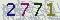﻿ 数学学院珠峰讲坛第605期：Minimum non-chromatic λ-choosable graphs-山东大学新闻网### 数学学院珠峰讲坛第605期：Minimum non-chromatic λ-choosable graphs

Minimum non-chromatic λ-choosable graphs

For a positive integer k, let f(k)=min{|V(G)|:χ(G)=k<ch(G)}. It was conjectured by Ohba, and proved by Noel, Reed and Wu that f(k)≥2k+2. This bound is sharp if k is even. Noel conjectured that if k is odd, then f(k)=2k+3. We proved this conjecture and hence determined the value of f(k) for all k. Assumeλ={k_1,…k_q} is a set of positive integers. Let k_λ=k_1+⋯+k_q. Aλ-list assignment of a graph G is a k_λ-list assignment L of G such that the set∪_(v∈V(G) ) L(v) of colours is partitioned into C_1∪…∪C_q and for each vertex v, |L(v)∩C_i |=k_i. We say G isλ-choosable if G is L-colourable for everyλ-list assignment L. The concept ofλ-choosability of graphs puts k-choosable and k-colourable in a same framework: Ifλ={1,…1} consists of k copies of 1, thenλ-choosable is equivalent to k-colourable. Ifλ={k}, thenλ-choosable is the same as k-choosable. For other partitionsλof k sandwiched between these two extremal partitions,λ-choosability reveals a complex hierarchy of colourabilities of graphs. Letλ=min{|V(G)|:χ(G)=k_λ}, G is notλ-choosable. We determined the value of f(λ) for allλ. This talk explains these results and some ideas in the proofs.

3月29日（周三）10:00-11:00

Zoom线上会议

【作者：张志越    来自：数学学院    编辑：新闻网工作室    责任编辑：强巴曲珍 蒋晓涵 　】

 验证码看不清楚,换张图片 共0条评论    共1页   当前第1页 拖动光标可翻页查看更多评论